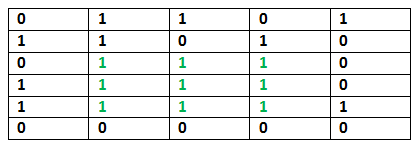# C Program for Maximum size square sub-matrix with all 1s

Given a binary matrix, find out the maximum size square sub-matrix with all 1s.

For example, consider the below binary matrix.## C/C++

 `// C/C++ code for Maximum size square ` `// sub-matrix with all 1s ` `#include ` `#define bool int ` `#define R 6 ` `#define C 5 ` ` `  `/* UTILITY FUNCTIONS */` `/* Function to get minimum of three values */` `int` `min(``int` `a, ``int` `b, ``int` `c) ` `{ ` `    ``int` `m = a; ` `    ``if` `(m > b) ` `        ``m = b; ` `    ``if` `(m > c) ` `        ``m = c; ` `    ``return` `m; ` `} ` ` `  `void` `printMaxSubSquare(``bool` `M[R][C]) ` `{ ` `    ``int` `i, j; ` `    ``int` `S[R][C]; ` `    ``int` `max_of_s, max_i, max_j; ` ` `  `    ``/* Set first column of S[][]*/` `    ``for` `(i = 0; i < R; i++) ` `        ``S[i] = M[i]; ` ` `  `    ``/* Set first row of S[][]*/` `    ``for` `(j = 0; j < C; j++) ` `        ``S[j] = M[j]; ` ` `  `    ``/* Construct other entries of S[][]*/` `    ``for` `(i = 1; i < R; i++) { ` `        ``for` `(j = 1; j < C; j++) { ` `            ``if` `(M[i][j] == 1) ` `                ``S[i][j] = min(S[i][j - 1], S[i - 1][j], ` `                              ``S[i - 1][j - 1]) ` `                          ``+ 1; ` `            ``else` `                ``S[i][j] = 0; ` `        ``} ` `    ``} ` ` `  `    ``/* Find the maximum entry, and indexes of maximum entry  ` `    ``in S[][] */` `    ``max_of_s = S; ` `    ``max_i = 0; ` `    ``max_j = 0; ` `    ``for` `(i = 0; i < R; i++) { ` `        ``for` `(j = 0; j < C; j++) { ` `            ``if` `(max_of_s < S[i][j]) { ` `                ``max_of_s = S[i][j]; ` `                ``max_i = i; ` `                ``max_j = j; ` `            ``} ` `        ``} ` `    ``} ` ` `  `    ``printf``(``"Maximum size sub-matrix is: \n"``); ` `    ``for` `(i = max_i; i > max_i - max_of_s; i--) { ` `        ``for` `(j = max_j; j > max_j - max_of_s; j--) { ` `            ``printf``(``"%d "``, M[i][j]); ` `        ``} ` `        ``printf``(``"\n"``); ` `    ``} ` `} ` ` `  ` `  ` `  `/* Driver function to test above functions */` `int` `main() ` `{ ` `    ``bool` `M[R][C] = { { 0, 1, 1, 0, 1 }, ` `                     ``{ 1, 1, 0, 1, 0 }, ` `                     ``{ 0, 1, 1, 1, 0 }, ` `                     ``{ 1, 1, 1, 1, 0 }, ` `                     ``{ 1, 1, 1, 1, 1 }, ` `                     ``{ 0, 0, 0, 0, 0 } }; ` ` `  `    ``printMaxSubSquare(M); ` `    ``getchar``(); ` `} `

Output:

```Maximum size sub-matrix is:
1 1 1
1 1 1
1 1 1
```

Please refer complete article on Maximum size square sub-matrix with all 1s for more details!

My Personal Notes arrow_drop_up
Article Tags :

Be the First to upvote.

Please write to us at contribute@geeksforgeeks.org to report any issue with the above content.